# Simple linear regression with online updates

Simple linear regression is a particular case of linear regression where we assume that the output $$y \in \mathbb{R}$$ is an affine transform of the input $$x \in \mathbb{R}$$:

\begin{equation*} y = \alpha + \beta x \end{equation*}

We gather observations $$(x_i, y_i)$$ and search for the parameters $$(\alpha, \beta)$$ that minimize a loss function such as the residual sum of squares. Graphically, we are fitting the line that minimizes the sum of squared distances to every point: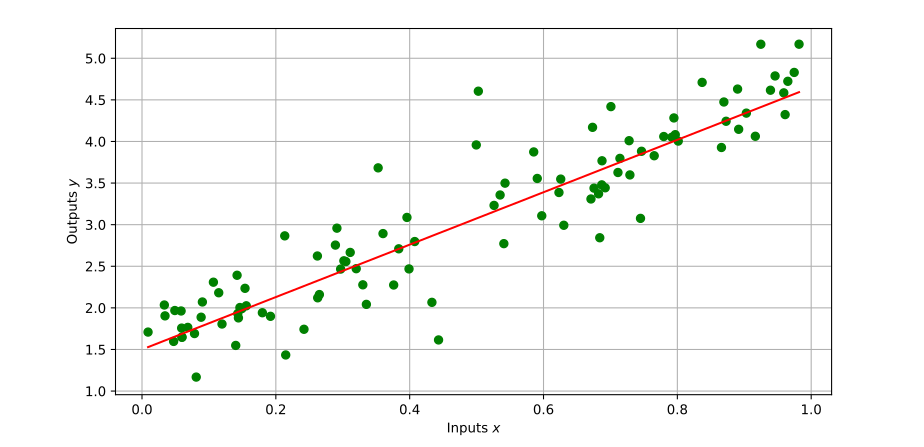Compared to a full-fledged linear regression, simple linear regression has a number of properties we can use for faster computations and online updates. In this post, we will see how to implement it in Python, first with a fixed dataset, then with online updates, and finally with a sliding-window variant where recent observations have more weight than older ones.

## Linear regression

Let us start with a vanilla solution using an ordinary linear regression solver. We stack our inputs into a vector $$\bfx = [x_1, \ldots, x_N]^\top$$ and their corresponding outputs into $$\bfy = [y_1, \ldots, y_N]^\top$$. Our objective function is the residual sum of squares:

\begin{equation*} \underset{\alpha, \beta}{\mathrm{minimize}} \ \| \bfy - \alpha \bfone - \beta \bfx \|_2^2 \end{equation*}

where $$\bfone$$ is the column vector filled with ones that has the same dimension as $$\bfx$$ and $$\bfy$$. The parameter $$\alpha$$ is called the intercept while $$\beta$$ is the slope (or coefficient).

### Using a machine learning library

For our tests, we will generate data using the same model as for the figure above:

import numpy as np

def generate_dataset(size=10000, alpha=1.5, beta=3.15):
x = np.random.random(size)
y = alpha + beta * x + np.random.normal(0, 0.4, size=x.shape)
return (x, y)


Any machine learning library will happily estimate the intercept and coefficients of a linear model from such observation data. Try it out with your favorite one! Here is for example how linear regression goes with scikit-learn:

import sklearn.linear_model

def simple_linear_regression_sklearn(x, y):
regressor = sklearn.linear_model.LinearRegression()
regressor.fit(x.reshape(-1, 1), y)
intercept = regressor.intercept_
slope = regressor.coef_
return (intercept, slope)


Yet, our problem is simpler than a general linear regression. What if we solved the least squares problem directly?

### Using a least squares solver

We can rewrite our objective function in matrix form as:

\begin{equation*} \| \bfy - \alpha \bfone - \beta \bfx \|_2^2 = \left\| \begin{bmatrix} \bfone & \bfx \end{bmatrix} \begin{bmatrix} \alpha \\ \beta \end{bmatrix} - \bfy \right\|_2^2 = \| \bfR \bfx - \bfy \|_2^2 \end{equation*}

with $$\bfR$$ the $$2 \times N$$ matrix with a first column of ones and $$\bfx$$ as second column. We can give these matrices to any least squares solver, for instance in Python using the solve_ls function from qpsolvers:

import qpsolvers

shape = (x.shape, 1)
R = np.hstack([np.ones(shape), x.reshape(shape)])
intercept, slope = qpsolvers.solve_ls(R, s=y, solver=solver)
return (intercept, slope)


This results in a faster implementation than the previous one, for instance on my machine:

In : x, y = generate_dataset(1000 * 1000)

In : %timeit simple_linear_regression_sklearn(x, y)
21.2 ms ± 22.8 µs per loop (mean ± std. dev. of 7 runs, 10 loops each)

In : %timeit simple_linear_regression_ls(x, y, solver="quadprog")
11.4 ms ± 73.9 µs per loop (mean ± std. dev. of 7 runs, 100 loops each)


The improvement may be due to avoiding extra conversions inside scikit-learn, or because the solver it uses internally is less efficient than quadprog for this type of problems. It is more significant for small datasets, and ultimately settles around $$2\times$$ for large datasets. Yet, we are still relying on a general least squares solution (we can add columns to $$\bfR$$ for more input variables), which means we are not squeezing out all the improvements we can get from simple linear regression.

## Simple linear regression

Let us expand our objective function using the vector dot product:

\begin{align} & \| \bfy - (\alpha \bfone + \beta \bfx) \|_2^2 \\ & = ((\alpha \bfone + \beta \bfx) - \bfy) \cdot ((\alpha \bfone + \beta \bfx) - \bfy) \\ & = \| \alpha \bfone + \beta \bfx \|_2^2 - 2 \bfy \cdot (\alpha \bfone + \beta \bfx) + (\bfy \cdot \bfy) \\ & = \alpha^2 (\bfone \cdot \bfone) + 2 \alpha \beta (\bfone \cdot \bfx) + \beta^2 (\bfx \cdot \bfx) - 2 (\bfone \cdot \bfy) \alpha - 2 (\bfx \cdot \bfy) \beta + (\bfy \cdot \bfy) \end{align}

Since this function is strictly convex, its global minimum is the (unique) critical point where its gradient vanishes:

\begin{align} \frac{\partial}{\partial \alpha} \| \bfy - (\alpha \bfone + \beta \bfx) \|_2^2 & = 0 & \frac{\partial}{\partial \beta} \| \bfy - (\alpha \bfone + \beta \bfx) \|_2^2 & = 0 \end{align}

Using the dot product expansion, this condition becomes:

\begin{align} 2 (\bfone \cdot \bfone) \alpha + 2 (\bfone \cdot \bfx) \beta + 0 - 2 (\bfone \cdot \bfy) + 0 + 0 & = 0 \\ 0 + 2 (\bfone \cdot \bfx) \alpha + 2 (\bfx \cdot \bfx) \beta - 0 - 2 (\bfx \cdot \bfy) + 0 & = 0 \end{align}

We end up with a $$2 \times 2$$ system of linear equations:

\begin{align} (\bfone \cdot \bfone) \alpha + (\bfone \cdot \bfx) \beta & = (\bfone \cdot \bfy) \\ (\bfone \cdot \bfx) \alpha + (\bfx \cdot \bfx) \beta & = (\bfx \cdot \bfy) \end{align}

We know its analytical solution by (manual Gaussian elimination or remembering the formula for) Cramer's rule:

\begin{align} \alpha & = \frac{(\bfone \cdot \bfy) (\bfx \cdot \bfx) - (\bfx \cdot \bfy) (\bfone \cdot \bfx)}{(\bfone \cdot \bfone) (\bfx \cdot \bfx) - (\bfone \cdot \bfx)^2} & \beta & = \frac{(\bfone \cdot \bfone) (\bfx \cdot \bfy) - (\bfone \cdot \bfx) (\bfone \cdot \bfy)}{(\bfone \cdot \bfone) (\bfx \cdot \bfx) - (\bfone \cdot \bfx)^2} \end{align}

The numerators and denominator of these two expressions have a statistical interpretation detailed in fitting the regression line (Wikipedia). The denominator is positive by the Cauchy-Schwarz inequality. It can be zero in the degenerate case where $$\bfx = x \bfone$$, that is, when all input values are equal, but we will assume that's not the case in what follows. All in all, the Python code corresponding to this solution needs only NumPy and floating-point arithmetic:

def simple_linear_regression_cramer(x, y):
dot_1_1 = x.shape  # == np.dot(ones, ones)
dot_1_x = x.sum()  # == np.dot(x, ones)
dot_1_y = y.sum()  # == np.dot(y, ones)
dot_x_x = np.dot(x, x)
dot_x_y = np.dot(x, y)
det = dot_1_1 * dot_x_x - dot_1_x ** 2  # not checking det == 0
intercept = (dot_1_y * dot_x_x - dot_x_y * dot_1_x) / det
slope = (dot_1_1 * dot_x_y - dot_1_x * dot_1_y) / det
return intercept, slope


This implementation is not only faster than the previous ones, it's also the first one where we leveraged some particular structure of our problem:

In : x, y = generate_dataset(1000 * 1000)

In : %timeit simple_linear_regression_ls(x, y, solver="quadprog")
11.4 ms ± 36 µs per loop (mean ± std. dev. of 7 runs, 100 loops each)

In : %timeit simple_linear_regression_cramer(x, y)
3.72 ms ± 160 µs per loop (mean ± std. dev. of 7 runs, 100 loops each)


We can use some IPython magic to compare the three approaches we have seen so far in a quick benchmark:

sizes = sorted([k * 10 ** d for k in [2, 5, 10] for d in range(6)])
for size in sizes:
x, y = generate_dataset(size)
instructions = [
"simple_linear_regression_sklearn(x, y)",
"simple_linear_regression_cramer(x, y)",
]
for instruction in instructions:
print(instruction)  # helps manual labor to gather the numbers ;)
get_ipython().magic(f"timeit {instruction}")


Here is the outcome in a plot (raw data in the image's alt attribute):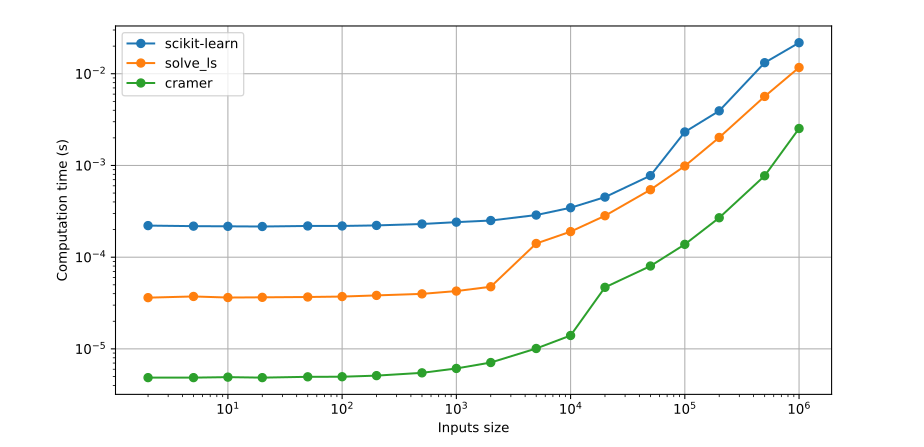We now have a reasonably efficient solution for simple linear regression over small- to medium-sized (millions of entries) datasets. All the solutions we have seen so far have been offline, meaning the dataset was generated then processed all at once. Let us now turn to the online case, where new observations $$(x_i, y_i)$$ come one after the other.

## Online simple linear regression

The dot products we use to calculate the intercept $$\alpha$$ and slope $$\beta$$ are ready for recursive updates. Suppose we already now their values for $$k$$ previous observations. When a new observation $$(x_{k+1}, y_{k+1})$$ comes in, we can update them by:

\begin{align} (\bfone \cdot \bfone)_{k+1} & = (\bfone \cdot \bfone)_k + 1 \\ (\bfone \cdot \bfx)_{k+1} & = (\bfone \cdot \bfx)_k + x_{k+1} \\ (\bfone \cdot \bfy)_{k+1} & = (\bfone \cdot \bfy)_k + y_{k+1} \\ (\bfx \cdot \bfx)_{k+1} & = (\bfx \cdot \bfx)_k + x_{k+1}^2 \\ (\bfx \cdot \bfy)_{k+1} & = (\bfx \cdot \bfy)_k + x_{k+1} y_{k+1} \end{align}

The same logic generalizes to a new batch of observations $$(\bfx_{k+1}, \bfy_{k+1})$$:

\begin{align} (\bfone \cdot \bfone)_{k+1} & = (\bfone \cdot \bfone)_k + \mathrm{dim}(\bfx_{k+1}) \\ (\bfone \cdot \bfx)_{k+1} & = (\bfone \cdot \bfx)_k + (\bfone \cdot \bfx_{k+1}) \\ (\bfone \cdot \bfy)_{k+1} & = (\bfone \cdot \bfy)_k + (\bfone \cdot \bfy_{k+1}) \\ (\bfx \cdot \bfx)_{k+1} & = (\bfx \cdot \bfx)_k + (\bfx_{k+1} \cdot \bfx_{k+1}) \\ (\bfx \cdot \bfy)_{k+1} & = (\bfx \cdot \bfy)_k + (\bfx_{k+1} \cdot \bfy_{k+1}) \end{align}

Keep in mind that, although we write them in vector form, dot products by $$\bfone$$ are simply sums. We can wrap these update rules in a Python class for a simple linear regressor that keeps track of dot products internally, and outputs $$\alpha$$ and $$\beta$$ upon new observations:

class SimpleLinearRegressor(object):
def __init__(self):
self.dots = np.zeros(5)
self.intercept = None
self.slope = None

def update(self, x: np.ndarray, y: np.ndarray):
self.dots += np.array(
[
x.shape,
x.sum(),
y.sum(),
np.dot(x, x),
np.dot(x, y),
]
)
size, sum_x, sum_y, sum_xx, sum_xy = self.dots
det = size * sum_xx - sum_x ** 2
if det > 1e-10:  # determinant may be zero initially
self.intercept = (sum_xx * sum_y - sum_xy * sum_x) / det
self.slope = (sum_xy * size - sum_x * sum_y) / det


This solution is equivalent to this answer from StackOverflow. It treats all observations the same, which is pertinent for non-time series data. But we can also tweak our derivation so far to the more specific use case of sliding-window regression over time series data.

## Sliding-window simple linear regression

Suppose now that single observations $$(x_i, y_i)$$ come in succession every $$\delta t$$ timesteps, and we want to estimate the instantaneous slope $$\beta$$ and intercept $$\alpha$$ of our recent data. Similarly to the exponential moving average filter, we can define a forgetting factor $$\lambda \in (0, 1)$$ as:

\begin{equation*} \lambda = \exp\left(-\frac{\delta t}{T}\right) \end{equation*}

where $$T$$ is the time constant that characterizes the span of our sliding window. Note that, by the Nyquist–Shannon sampling theorem, $$T$$ has to be at least $$2 \delta t$$ to avoid aliasing. We can use this forgetting factor in our objective function as a discount rate applied to previous observations:

\begin{equation*} \mathit{fit}(\alpha, \beta, \bfx_k, \bfy_k) = \sum_{i=0}^{k-1} \lambda^{i} (y_{k-i} - \alpha - \beta x_{k-i})^2 \end{equation*}

To calculate this exponentially discounted residual sum of squares efficiently, we can modify the definition of our vectors $$\bfx_k$$, $$\bfy_k$$, and $$\bfone_k$$, to incorporate the forgetting factor:

\begin{align} \bfx_{k+1} & = \begin{bmatrix} \sqrt{\lambda} \bfx_k \\ x_{k+1} \end{bmatrix} & \bfy_{k+1} & = \begin{bmatrix} \sqrt{\lambda} \bfy_k \\ y_{k+1} \end{bmatrix} & \bfone_{k+1} & = \begin{bmatrix} \sqrt{\lambda} \bfone_k \\ 1 \end{bmatrix} \end{align}

The benefit of this approach is that the squared norms of our new vectors now correspond to the exponentially discounted residual sum of squares:

\begin{align} \| \bfy_{k+1} - \alpha \bfone_{k+1} - \beta \bfx_{k+1} \|_2^2 & = (y_{k+1} - \alpha - \beta x_{k+1})^2 + \lambda \| \bfy_{k} - \alpha \bfone_k - \beta \bfx_k \|_2^2 \\ & = \ \ldots \\ & = \sum_{i=0}^{k} \lambda^{i} (y_{k+1-i} - \alpha - \beta x_{k+1-i})^2 \\ & = \mathit{fit}(\alpha, \beta, \bfx_{k+1}, \bfy_{k+1}) \end{align}

Granted, we took some liberties with the notation $$\bfone_k$$ as it's not a vector of ones any more ;-) But the expression $$(\bfone_k \cdot \bfv_k)$$ keeps the semantic of "summing the coordinates of $$\bfv_k$$", although is now in an exponentially discounted sense. In terms of dot products, our update rules translate to:

\begin{align} (\bfone \cdot \bfone)_{k+1} & = \lambda (\bfone \cdot \bfone)_k + 1 \\ (\bfone \cdot \bfx)_{k+1} & = \lambda (\bfone \cdot \bfx)_k + x_{k+1} \\ (\bfone \cdot \bfy)_{k+1} & = \lambda (\bfone \cdot \bfy)_k + y_{k+1} \\ (\bfx \cdot \bfx)_{k+1} & = \lambda (\bfx \cdot \bfx)_k + x_{k+1}^2 \\ (\bfx \cdot \bfy)_{k+1} & = \lambda (\bfx \cdot \bfy)_k + x_{k+1} y_{k+1} \end{align}

This leads to a regressor class similar to the previous one:

class SlidingWindowLinearRegressor(object):
def __init__(self, dt, time_constant):
self.dots = np.zeros(5)
self.intercept = None
self.forgetting_factor = np.exp(-dt / time_constant)
self.slope = None

def update(self, x: float, y: float):
self.dots *= self.forgetting_factor
self.dots += np.array([1, x, y, x * x, x * y])
dot_11, dot_1x, dot_1y, dot_xx, dot_xy = self.dots
det = dot_11 * dot_xx - dot_1x ** 2
if det > 1e-10:  # determinant is zero initially
self.intercept = (dot_xx * dot_1y - dot_xy * dot_1x) / det
self.slope = (dot_xy * dot_11 - dot_1x * dot_1y) / det


Let's evaluate its performance on synthetic data:

dt = 1e-3  # [s]
T = 5e-2  # [s]
sliding_window_regressor = SlidingWindowLinearRegressor(dt, T)
for t in np.arange(0., 100., dt):
alpha = 1.5 * np.sin(0.1 * t)
beta = 3.15 + 0.5 * np.cos(1.1 * t)
x = np.cos(t) + np.random.normal(0, 0.05)
y = alpha + beta * x + np.random.normal(0, 0.1)
sliding_window_regressor.update(x, y)


This small test results in the following observations and estimates: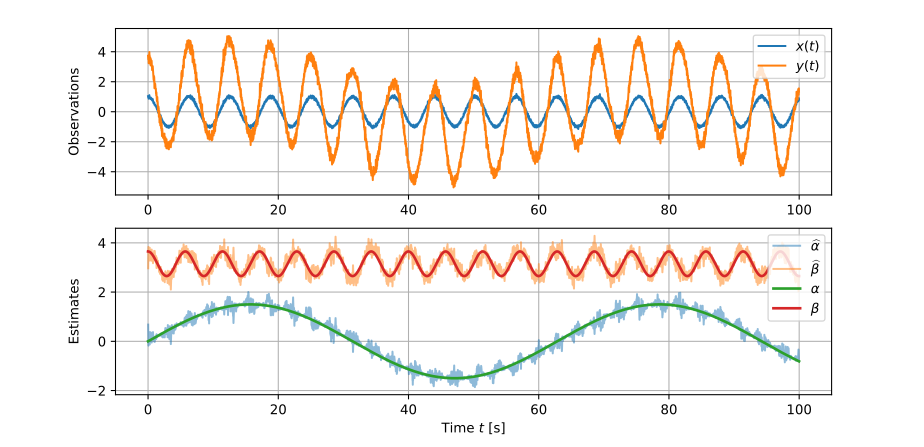Contrary to regular simple linear regression, our filter now has a time-constant parameter $$T$$ that defines the length of our sliding window. Its ideal value depends on our data. In this example, it would depend on all the inline parameters [1.5, 0.1, 3.15, 0.5, 1.1, 0.05, 0.1] we use to produce $$\alpha(t), \beta(t)$$ and the white noise on $$x(t), y(t)$$. Although, mainly, it will depend on the two constants [0.1, 1.1] that define how fast $$\alpha(t)$$ and $$\beta(t)$$ change. If we choose it too small, our estimates will be snappy but noisy. For example with T = 5e-3 s: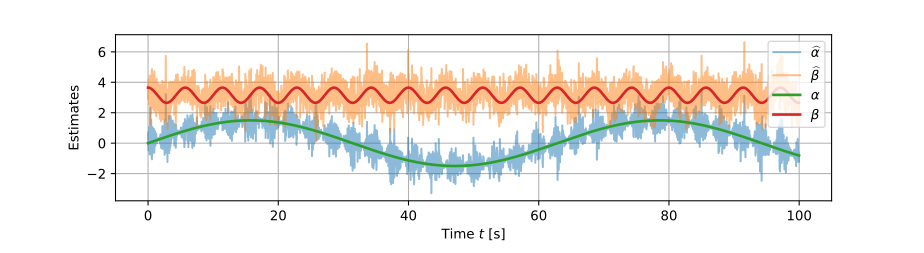If we choose it too large, our estimates will be smooth but delayed. For example with T = 5.0 s: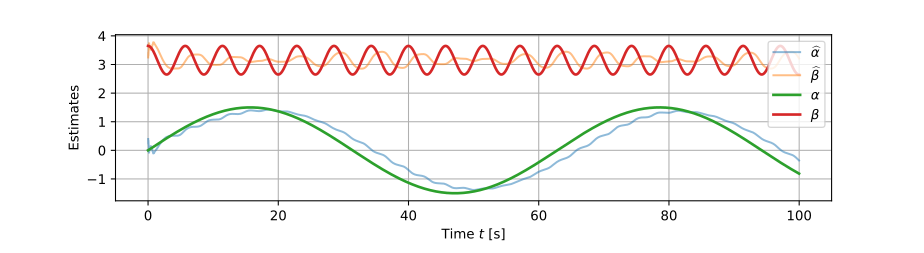In this instance, the filter may be acceptable for $$\alpha(t)$$ but it fails to capture the time variations of $$\beta(t)$$ (if we crank $$T$$ up further we can even average them out completely). How to tune it from there depends on the desired behavior.

## To go further

Sliding window linear regression combines statistics and signal processing. Its parameter $$T$$ is the same time constant as in a low-pass filter, and it can be selected by specifying a frequency response, which does formally what we commented on informally in our example when selecting $$T$$ to "capture" or not the time-varying dynamics of $$\alpha(t)$$ and $$\beta(t)$$. The overall algorithm itself is a simplification of the recursive least squares filter to our use case.

When deriving our system of linear inequalities for offline regression, the condition we used that the gradient vanishes at the global minimum of the objective function is a particular case of Karush–Kuhn–Tucker (KKT) conditions applied to our unconstrained problem. KKT conditions provide the general solution when the problem also has inequality or equality constraints.

### Bonus: simple linear regression with JAX

For fun, we can look at the performance of the offline algorithm using JAX's just-in-time compiler. JAX also gives us a convenient way to try running the function on the CPU or GPU, so let's try both:

import jax
import jax.numpy as jnp

def simple_linear_regression_cramer_jax(x: jnp.array, y: jnp.array):
dot_1_1 = x.shape  # == jnp.dot(ones, ones)
dot_1_x = x.sum()  # == jnp.dot(x, ones)
dot_1_y = y.sum()  # == jnp.dot(y, ones)
dot_x_x = jnp.dot(x, x)
dot_x_y = jnp.dot(x, y)
det = dot_1_1 * dot_x_x - dot_1_x ** 2  # not checking det == 0
intercept = (dot_1_y * dot_x_x - dot_x_y * dot_1_x) / det
slope = (dot_1_1 * dot_x_y - dot_1_x * dot_1_y) / det
return intercept, slope

cpu = jax.devices("cpu")
gpu = jax.devices("gpu")
cramer_jax_cpu = jax.jit(simple_linear_regression_cramer_jax, device=cpu)
cramer_jax_gpu = jax.jit(simple_linear_regression_cramer_jax, device=gpu)

for size in sizes:
x, y = generate_dataset(size)
jx, jy = jnp.array(x), jnp.array(y)
instructions = ["cramer_jax_cpu(jx, jy)", "cramer_jax_gpu(jx, jy)"]
for instruction in instructions:
get_ipython().magic(f"timeit {instruction}")


Here are the results of this benchmark on my machine (CPU: Intel® Core™ i7-6700K at 4.00 GHz, GPU: NVIDIA GeForce GTX 1070 with CUDA 10.1), combined with the ones from the previous benchmark above: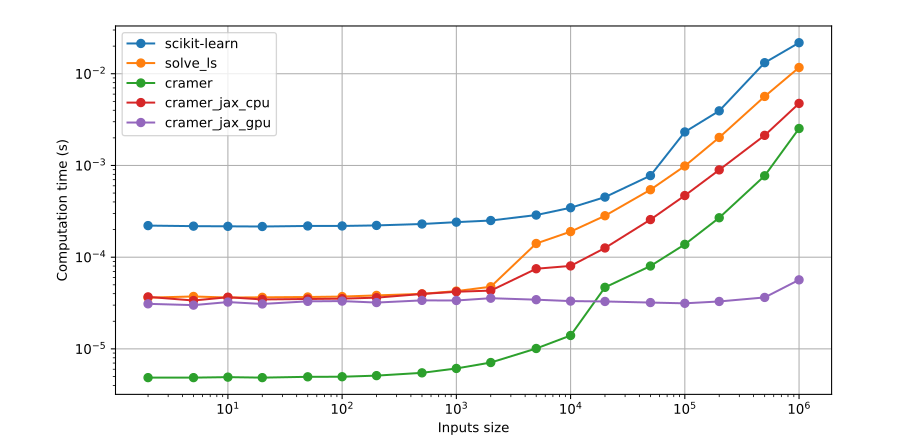Nothing beats simple NumPy on small datasets, and the turning point when transferring to GPU becomes attractive seems to be around 20,000 observations.

## Discussion

You can use Markdown with $\LaTeX$ formulas in your comment.